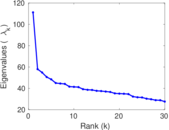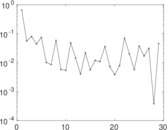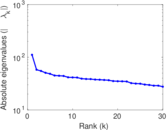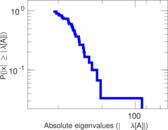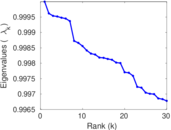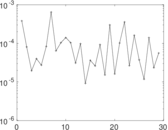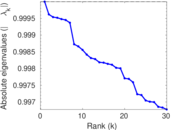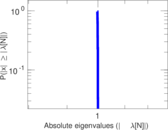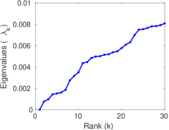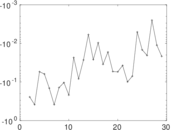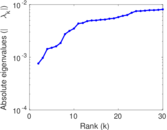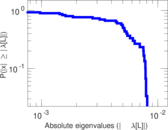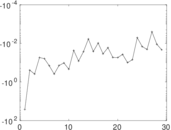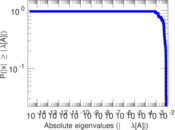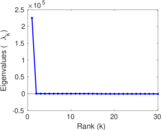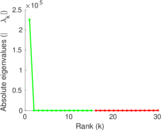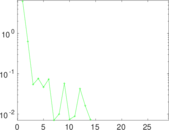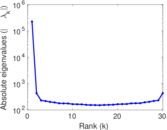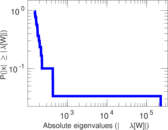# DBpedia locations

This is the bipartite network of entities in Wikipedia and their locations. The nodes of the network are entities and locations. Edges denote that an entity is associated with a location. Entity can have multiple locations, for instance cities associated with a company. The edges correspond to the <http://dbpedia.org/ontology/location> relationships in DBpedia.

 Code `LO` Internal name `dbpedia-location` Name DBpedia locations Data source http://wiki.dbpedia.org/Downloads AvailabilityDataset is available for download Consistency checkDataset passed all tests Category Feature network Dataset timestamp 2001 ⋯ 2017 Node meaning Entity, place Edge meaning Location Network formatBipartite, undirected Edge typeUnweighted, no multiple edges

## Statistics

 Size n = 225,498 Left size n1 = 172,091 Right size n2 = 53,407 Volume m = 293,697 Wedge count s = 122,892,989 Claw count z = 324,552,882,549 Cross count x = 929,789,499,617,832 Square count q = 3,761,594 4-Tour count T4 = 522,254,610 Maximum degree dmax = 12,189 Maximum left degree d1max = 28 Maximum right degree d2max = 12,189 Average degree d = 2.604 87 Average left degree d1 = 1.706 64 Average right degree d2 = 5.499 22 Fill p = 3.195 53 × 10−5 Size of LCC N = 181,937 Diameter δ = 27 50-Percentile effective diameter δ0.5 = 5.948 20 90-Percentile effective diameter δ0.9 = 8.770 09 Median distance δM = 6 Mean distance δm = 6.754 43 Gini coefficient G = 0.540 934 Balanced inequality ratio P = 0.305 049 Left balanced inequality ratio P1 = 0.404 226 Right balanced inequality ratio P2 = 0.187 612 Relative edge distribution entropy Her = 0.888 942 Power law exponent γ = 3.176 22 Tail power law exponent γt = 2.011 00 Tail power law exponent with p γ3 = 2.011 00 p-value p = 0.000 00 Left tail power law exponent with p γ3,1 = 6.221 00 Left p-value p1 = 0.000 00 Right tail power law exponent with p γ3,2 = 2.021 00 Right p-value p2 = 0.000 00 Degree assortativity ρ = +0.082 583 3 Degree assortativity p-value pρ = 0.000 00 Spectral norm α = 111.155 Algebraic connectivity a = 0.000 760 771 Spectral separation |λ1[A] / λ2[A]| = 1.917 68 Controllability C = 124,316 Relative controllability Cr = 0.551 325

## Plots

### Fruchterman–Reingold graph drawing### Degree distribution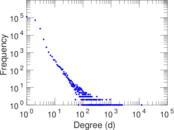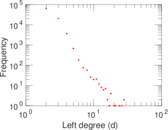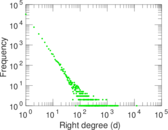### Cumulative degree distribution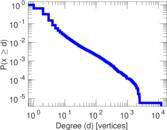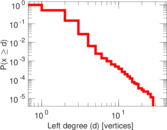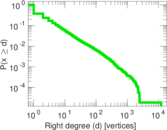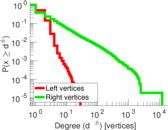### Lorenz curve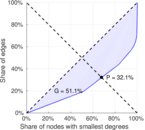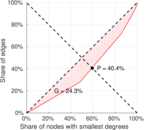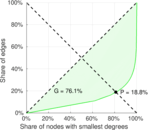### Spectral distribution of the adjacency matrix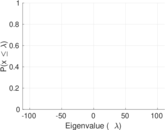### Spectral distribution of the normalized adjacency matrix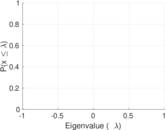### Spectral distribution of the Laplacian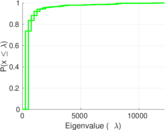### Spectral graph drawing based on the adjacency matrix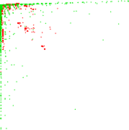### Spectral graph drawing based on the Laplacian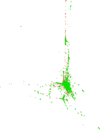### Spectral graph drawing based on the normalized adjacency matrix### Degree assortativity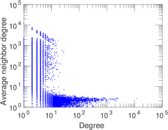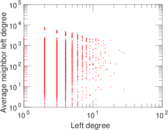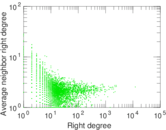### Zipf plot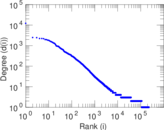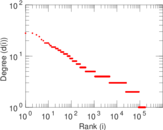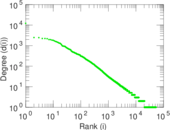### Hop distribution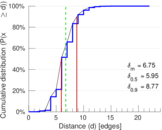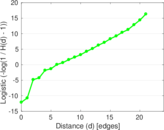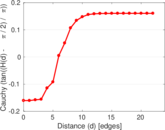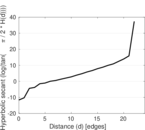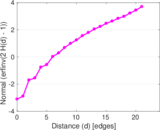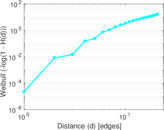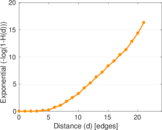### Double Laplacian graph drawing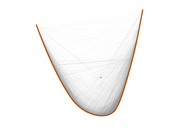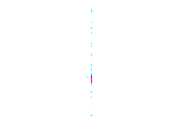### Delaunay graph drawing### Matrix decompositions plots